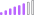cancel
Showing results for
Did you mean:Post Patron

## Per Unit Computation Dax

Hi Guys,

Would need your help in constructing a dax that computes the per unit computation. The computation of Per Unit is (Present value) ÷ (Maximum Data Point) I'm using a date hierarcy (Year, Quarter & Month) and other dimensions. So the maximum data point will depedend on the filters selected.

Expectation:

The maximum value for this is 999 (September) so we will divide each value by 999.

Example: 456/999 = 0.46

You can play with this Sample pbix: https://www.mediafire.com/file/0pbtogvc6kib02k/sample_powerbi.pbix/fileThank you so much in advance!!

1 ACCEPTED SOLUTIONCommunity Support

Hi @icdns ,

I download you sample and have test. Firstly I suggest to to sort [Month] column by [MonthNo] column.

For reference: Sort one column by another column in Power BI Desktop

Then create a measure as below to achieve your goal.

``````Sales Percentage =
VAR _SUMMARIZE =
SUMMARIZE (
ALLSELECTED ( sampledata ),
sampledata[Year],
sampledata[Month],
sampledata[City],
"Sales", CALCULATE ( SUM ( sampledata[Sales] ) )
)
_SUMMARIZE,
"MaxSales", MAXX ( FILTER ( _SUMMARIZE, [City] = EARLIER ( [City] ) ), [Sales] )
)
VAR _Sales =
CALCULATE ( SUM ( sampledata[Sales] ) )
VAR _MaxSales =
MAXX ( FILTER ( _ADDMAX, [City] = MAX ( sampledata[City] ) ), [MaxSales] )
RETURN
DIVIDE ( _Sales, _MaxSales )``````

Result is as below.Best Regards,
Rico Zhou

If this post helps, then please consider Accept it as the solution to help the other members find it more quickly.

5 REPLIES 5Post Patron

Thank you for this. It is now working when it's on a monthly view. However, in my table I have also Quarter and Year column. If I drill up to yearly and quarterly view the value adds up. (Ex. If the view is Yearly, the value point let say 2016 is the TOTAL per unit of the 12 months (Jan-Dec))

The computation must be the same on how we compute with the monthly view wherein (Present value) ÷ (Maximum Data Point)

How can we insert this in the dax? 🙂

Thanks!Community Support

Hi @icdns ,

I download you sample and have test. Firstly I suggest to to sort [Month] column by [MonthNo] column.

For reference: Sort one column by another column in Power BI Desktop

Then create a measure as below to achieve your goal.

``````Sales Percentage =
VAR _SUMMARIZE =
SUMMARIZE (
ALLSELECTED ( sampledata ),
sampledata[Year],
sampledata[Month],
sampledata[City],
"Sales", CALCULATE ( SUM ( sampledata[Sales] ) )
)
_SUMMARIZE,
"MaxSales", MAXX ( FILTER ( _SUMMARIZE, [City] = EARLIER ( [City] ) ), [Sales] )
)
VAR _Sales =
CALCULATE ( SUM ( sampledata[Sales] ) )
VAR _MaxSales =
MAXX ( FILTER ( _ADDMAX, [City] = MAX ( sampledata[City] ) ), [MaxSales] )
RETURN
DIVIDE ( _Sales, _MaxSales )``````

Result is as below.Best Regards,
Rico Zhou

If this post helps, then please consider Accept it as the solution to help the other members find it more quickly.Super User

Hi,

I hope you can get an idea on how to create a measure from the below picture and the attached pbix file.``````Per unit measure: =
VAR maxcount =
CALCULATE ( MAX ( Data[Count] ), REMOVEFILTERS ( Data ) )
RETURN
DIVIDE ( [Count measure:], maxcount )
``````

If this post helps, then please consider accepting it as the solution to help other members find it faster, and give a big thumbs up.Super User

@icdns , Assuming count is measure create a measure like this for denominatorPost Patron

Hi @amitchandak ,

that is what im planning to do. But the problem is i can't get the correct maxmium data point 😞